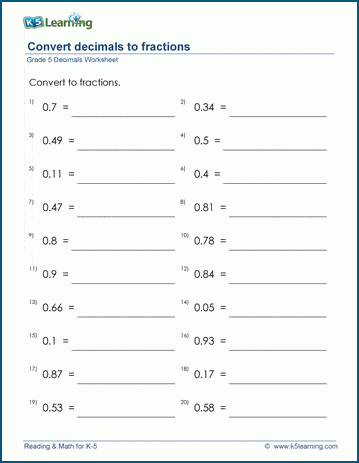# Convert decimals to fractions

## Math worksheets: Convert 1 or 2 digit decimals to fractions without simplifying

Below are six versions of our grade 5 math worksheet on converting simple decimal numbers to fractions with tens or hundreds as the denominator; students are not asked to simplify the answer. These worksheets are pdf files.## More fractions worksheets

Explore all of our fractions worksheets, from dividing shapes into "equal parts" to multiplying and dividing improper fractions and mixed numbers.

## More decimals worksheets

Find all of our decimals worksheets, from converting fractions to decimals to long division of multi-digit decimal numbers.

## What is K5?

K5 Learning offers reading and math worksheets, workbooks and an online reading and math program for kids in kindergarten to grade 5.  We help your children build good study habits and excel in school.